blog

# Put Option Profit Formula

#### Options Trading 101 - The Ultimate Beginners Guide To Options

As Seen On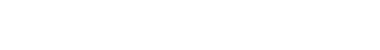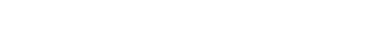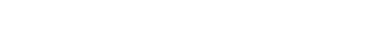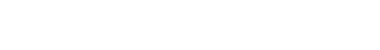by Gavin in BlogA put option is a contract that gives the owner the right, but not the obligation, to sell an agreed-upon number of an underlying security (e.g. shares), by a specific date and to sell the underlying security at a pre-determined price, called the strike price.

As the price of the underlying security changes, it will affect the price of the put option and thus the potential profit that the put holder can enjoy.

So how is a put option profit calculated?

In order to understand how profitable a put option is, you must first understand the concept of intrinsic value.

Intrinsic value is the difference between the current market price of the underlying asset and the strike price of the option.

Whenever the strike price of a put is greater than the market price of the underlying asset, it is considered to be in-the-money.

This is important to remember as only in-the-money options have an intrinsic value.

The second concept to understand is the time value of an option.

The time value of an option decays as the option approaches the expiration date.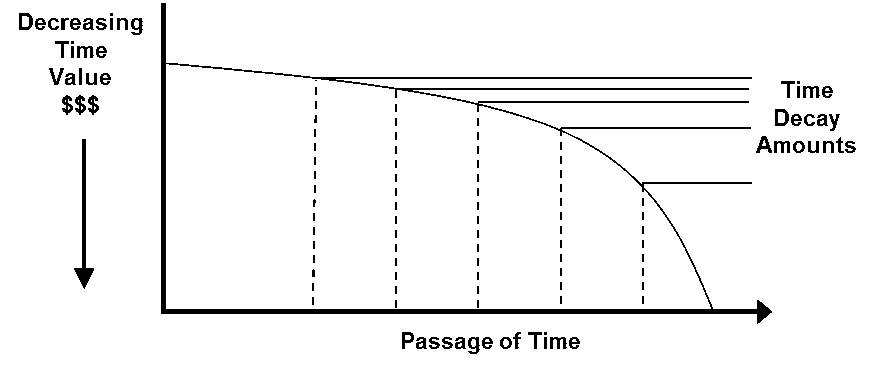Image credit: CME Group

What this means is that as the expiration date gets closer, the more the value of the option is attributed to the intrinsic value.

Finally, when the option expires, it has no time value component so its value is completely determined by the intrinsic value.

So how do you calculate the intrinsic value of an option?

We’ll demonstrate how, using a worked example.

Suppose you purchased a \$20 put option for company ABC at a strike price of \$75.

If the stock of ABC is currently trading at \$70, you would enjoy an intrinsic value of \$15.

This is calculated by taking the price of the put option (\$20) and subtracting the difference between the strike price and the current underlying price (\$75 – \$70 = \$5).

The remaining 5 points are simply the premium that is attributable to time value (which will decline as the option approaches expiration).

Since each option contract is for 100 shares, this means that the total cost of the put option would be \$2,000 (which is 100 shares x the \$20 purchase price).

In order to be profitable in this scenario, you would need the intrinsic value to be at least \$20 by the time the option reaches expiration.

Putting that all together, we can derive the profit formula for a put option:

Profit = (( Strike Price – Underlying Price ) – Initial Option Price ) x number of contracts

Using the previous data points, let’s say that the underlying price at expiration is \$50, so we get:

Profit = (( \$75 – \$50) – \$20) x 100 contracts

Profit = (( \$25 ) – \$20 ) x 100 contracts

Profit = \$5 x 100 contracts

Profit = \$500

Similarly, if the stock doesn’t decline as much (i.e. the intrinsic value is less than \$20) we will make a loss.

For example, if the price was instead \$65 at expiration, we would have the following:

Profit = (( \$75 – \$65) – \$20) x 100 contracts

Profit = (( \$10 ) – \$20 ) x 100 contracts

Profit = – \$10 x 100 contracts

Profit = – \$1000 (i.e. a loss of \$1,000)